## Order of Operations and Mentions:

###### Example
SMph. = √30D'f ± 1
D' = 120' and f = .8
Note: 30Df ±1 could look like (30Df ±1) or 30 * D * f ±1
SMph. = √30 * 120' * 0.8 ± 1

###### Applying (-) Negative and or (+) Positive Integers (Numbers).
What if, we have to apply a negative integer to a positive integer?
We need to subtract the negative integer from the positive integer:
- 1 ← Negative Integer + 1 ← Positive Integer = 0
- 1 ← Negative Integer + 2 ← Positive Integer + .5 ← Positive Integer = 1.5 ← Positive Integer
What if we have to apply a negative integer to a negative integer?
We need to add negative integers and the result is a negative integer:
- 1 ← Negative Integer + - 1 ← Negative Integer = - 2 ← Negative Integer.
- 1 ← Negative Integer + - 2 ← Negative Integer + -.05 ← Negative Integer = -3.05 ← Negative Integer.
SMph. = √2880 -1
We subtracted 1 or added -1
SMph. = 2879
Then the radical √ = square root
SMph. = 53.65631370118525###### ☝ Click image to enlargeConsider this, It is not necessary to know any derivation by heart, but it is necessary to know or have a very good understanding of what we are doing. When we apply our work to a profession environment (I.e. Court), remember we must do our work correctly (In other words; Check, check, and check again, then get someone else to check, check, and check again!), show the math, and clearly display our method used in getting our results in our explanation.Consider this, We need to and should take note of a conversion factor that we will use in nearly every formula derivation.

That is the number 1.466. This number is found by dividing the number of feet in a mile = 5280 feet' by the total number of seconds in an hour 3600 seconds(sec.). The result gives us 1.4666666, which we will to 1.466.

Note: We do not round any further and must us this result as is and 1.466 is used to convert (Feet per Second)fps. into (Miles Per Hour)Mph. or vice versa. As an example: 60 Mph. times 1.466 equals 88 fps. or 88 fps. divided by 1.466 equals 60 Mph..

We see Velocity (Vfps.), which is in fps., is replaced by (SMph. x 1.466), which changes to fps. to Mph. and we have not altered the equations.Consider this, we will see 1.6 which = 1.6 seconds(sec.) 1.6 secs. is a representation of a average reaction time that we as humans have when we have no other external or internal impeding stimulus effecting us.

If we don't remember any other thing, it is imperative that we remember this mention. If you don't understand it, contact someone who does and get some assistant and better understanding.
Ke = .05(M)V2 is the formula for energy in motionConsider this, We we see the numerical value 30 mentioned in expressions, 30 is a derivative. What are Derivatives? They are the result of performing a differentiation process upon a function or an expression. Derivative notation is the way we express derivatives mathematically.

## Legend (Key):

= Symbol for unit of measure Inches.
= Symbol for unit of measure Feet.
Ke = .05(M)V2 Kinetic Energy formula for energy of motion.
D = Distance in feet.
S = Speed in miles per hour (Mph.).
V = Velocity in feet per second (fps.).
f = Drag factor (Coefficient of Friction).
t = Time (In Seconds ≡ Sec.).
C = Chord (Feet ').
g = Acceleration of gravity or 32.22
feet per second squared (32.22 fps.).
H = Height (Feet ').
Sc = Combined Speed (Mph.).
W = Weight (lbs.) = How much gravity is pulling down on an object.
n = % Breaking Efficiency.

M = Mass = How much matter is contained within an object.
of an object divided by the acceleration of gravity or W/g.
30 = A constant that arises in the process of the derivation.
15 = ½ of the constant of 30 that arose in the process of the derivation.
1.466 = A conversion factor: 5280 '/3600 sec. in a hour.
1.6 sec. = A normal average reaction time in seconds.
μ = Coefficient of Friction on Level Surface
Note: Here for a level surface μ = f.
Se = Super elevation 2% would be converted to .02 ☚ More info.

Vo = Original Velocity (fps.).

## Expressions:

So, We have a mini van that weighs(W) 4000 lbs, traveling on a level surface, and as it travels it Kinetic Energy (F) is 3000 lbs. We need to find μ for our Coefficient of Friction (f).
μ = F ÷ W← Will work!

###### Click Image to enlarge

Open image in separate window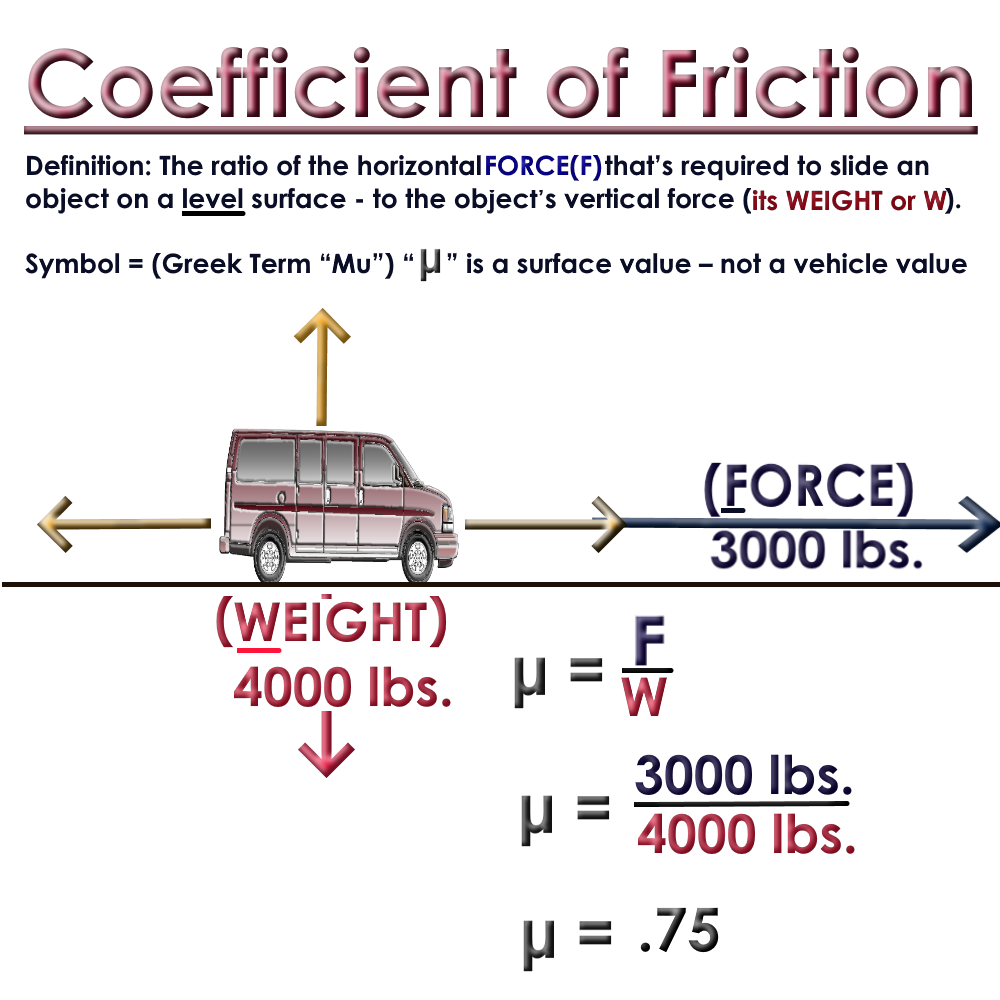We know that a vehicle skidding downhill will have a reduced(-) less coefficient of friction and a vehicle skidding uphill will have a greater(+) more coefficient of friction.Consider this, A mini van crash takes place where the road surface is Asphalt and we have a 2% downhill grade.

Using our Asphalt (Well Worn) has a Coefficient of Friction range of .45 - .75. Giving the benefit to the operator (Note: Use the higher decimal) We determined the Coefficient of Friction (μ) is .75 with a Downhill grade of 2%.
f = µ ± m← Will Work!

KNOWNS DOWNHILL:
µ = .75
f = -.02
RESULT:
f = µ ± m
f = .75 ± -.02 ← Downhill Grade we use negative(subtract).
f = 0.73
KNOWNS UPHILL:
µ = .75
f = -.02
RESULT:
f = µ ± m
f = 0.77
###### Click image to enlarge

Open image in separate window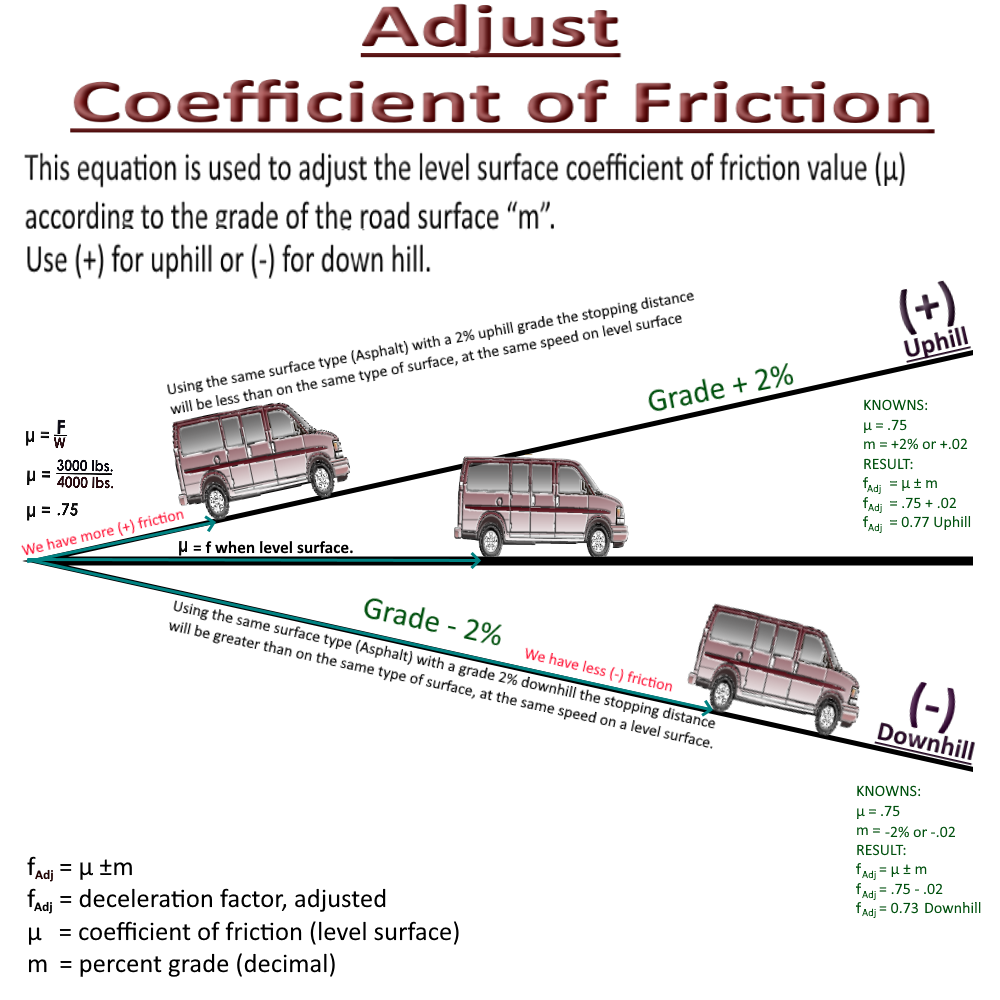Special Note: Finding f = μ ± m like other formulas listed are derived from a more complex algebraic formulas. Being familiar with these other formulas could help you visualize the mathematical progression.Consider this, So the above equations referred to the grade being level and the breaking system operating at 100%.

What if the, We determined only the front two breaks were working with vehicle involved in our collision and the vehicle was Front Wheel Drive?

We need to get the (n) breaking efficiency for our crash vehicle.

Note: If there is one brake (or more) that was not working on a vehicle, the coefficient of friction must be adjusted to represent the lack of (or diminished) braking efficiency on each wheel.

###### Click image to enlarge

Open image in separate window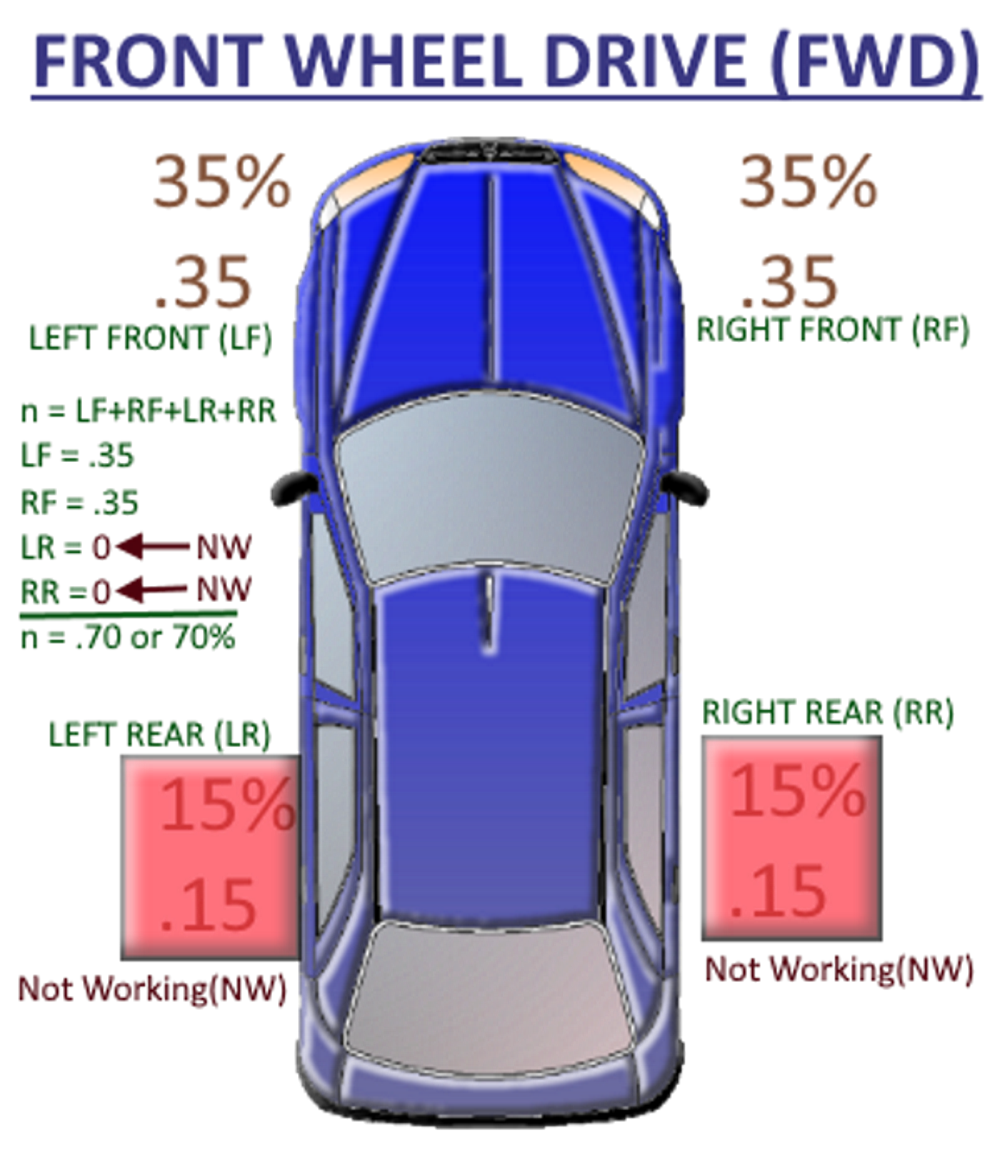We said the vehicle is FRONT WHEEL DRIVE (FWD) and according to Breaking Efficiency diagram above the LEFT FRONT(LF) tire provides 35% = .35 of the vehicles breaking and the RIGHT FRONT (RF) tire provides 35% = .35 of our vehicle's breaking, and the two rear tires (LR, RR) each provide 15% = .15, but the two rear breaks were Not Working(NW), so they are providing no breaking or 0% = .0.

So, we add 35% = .35 for the LEFT FRONT(LF) tire, 35% = .35 for the RIGHT FRONT(RF) tire, 0% = .0 for the LEFT REAR(LR) tire, and 0% = .0 for the RIGHT REAR(RR) tire.
(n) = Total Breaking Percentage or our vehicles Breaking Efficiency is 70% = .70

Special Note: Finding D = Skid Distance (D) like other formulas listed are derived from a more complex algebraic formulas. Being familiar with these other formulas could help you visualize the mathematical progression.Our Front Wheel Drive (FWD) vehicle is skidding on a surface (Asphalt) with diminished breaking efficiency due to only the front two breaks working. We also determined the rear breaks are Not Working(NW) at all, and we have a Uphill road grade of 2%.

We know that we have to adjust the Coefficient of Friction (f) when one or more breaks are not working, and we have to adjust the Coefficient of Friction (f) as well for the road grade (m).

Using our "f = Coefficients" chart the Coefficient of Friction (μ) for (Asphalt) level (Well Worn and Dry) we get a range of .60 - .75 . Giving the benefit to the operator we use the higher of the range (.75), then using our "n = Breaking Efficiency" chart we have the determined the braking efficiency (n) to have been at 70% or .7, and our Uphill(+) road grade elevation(m) to be 2% or .02
f = μ(n) ± m will work.

KNOWNS:
µ = .75
n = .7
m = .02
RESULT:
f = µ(n) ± m
f = .75(.7) ± .02
f = 0.525 ± .02
f = 0.545

###### Click image to enlarge

Open image in separate windowOpen image in separate windowSpecial Note: Finding f = μn ± m like other formulas listed are derived from a more complex algebraic formulas. Being familiar with these other formulas could help you visualize the mathematical progression.

Minimum Speed from Skid Marks formula - When using this formula we can derive the minimum speed of a vehicle from skid marks, again providing us with the vehicle's minimum speed.

Unlike critical speed which provides us with the exact speed of the vehicle, minimum speed is just that...The vehicles minimum speed where the skid marks begin.

Simply put, the vehicle could not possibly be going any slower than the calculated minimum speed, but the vehicle's actual speed might be faster.

So...If we have a vehicle that ends up in a collision with a another motor vehicle or a rigid object, and we have measured skid marks, and we assume the drag factor being constant with the road's grade and/or super elevation are level.
S = √30 (D)f will work.

###### Example

Open image in separate window

###### Click image to enlarge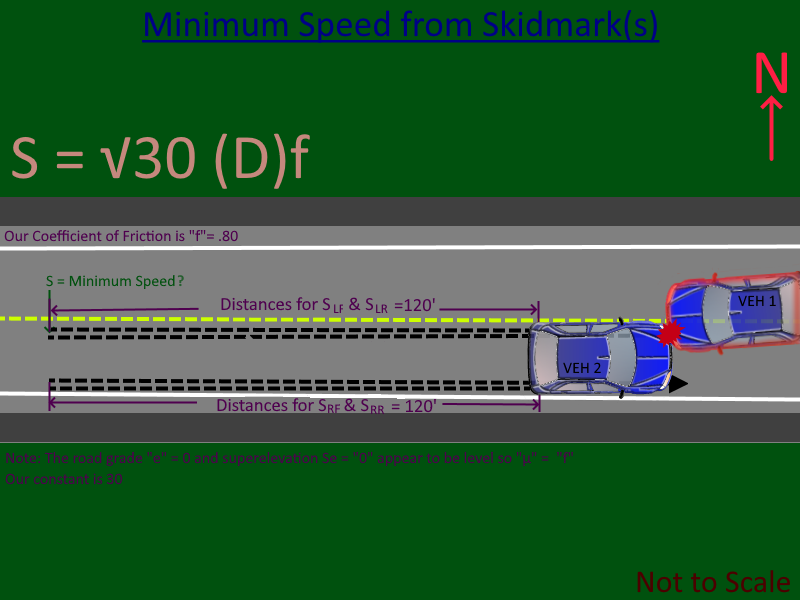DAvg. = S1 + S2 / Number of Skid Marks measured.
DAvg. = 120' + 120' / 2
DAvg. ≡ D = 120'

We've determined that the Asphalt has a Drag Factor of (f = .80). We've measured the tire skid marks and the Distance(D) of the skid marks is D = 120 feet'. Since all four tires were locked and skidding we've determined the vehicle breaking system is working with 100% breaking efficiency, also our road grade and super-elevations are "0".

S = √30 Df
S = √30 * 120' * 0.8
S = √3600' * 0.8
S = √2880'
S = 53.66 Mph ☚ The vehicles minimum speed where skid marks begin.Consider this, What if the same vehicle skids between different surfaces such as from asphalt, onto concrete, and then onto grass? The drag factors of all surfaces must be measured and included, and then used in the Combined Speed formula.
SC = √S2Asphalt + S2Concrete + S2GrassConsider this, What if in the same vehicle had the left side tires skidding on asphalt and the right side tires skidding on a different surface, lets say the noted grass?

We then utilize a speed formula derived by changing the constant = (30) to ½ that of the constant = (15) and then adding the two different drag factors, one for the Asphalt fAsphalt road for the left tires and the drag factor of the Grass fGrass surface for the right tires.
S = √15 * (fAsphalt + fGrass) * D

Special Note: The minimum speed formula from skid marks like other formulas listed are derived from a more complex algebraic formula. Being familiar with the derivation of the minimum speed formula could help you visualize the mathematical progression.

Consider this, What if in the same vehicle had the left side tires skidding on asphalt and the right side tires skidding on a different surface, lets say the noted grass?

We then utilize a speed formula derived by changing the constant = (30) to ½ that of the constant = (15) and then adding the two different drag factors, one for the Asphalt fAsphalt road for the left tires and the drag factor of the Grass fGrass surface for the right tires.
S = √15 * (fAsphalt + fGrass) * D

Special Note: Finding D = Skid Distance (D) like other formulas listed are derived from a more complex algebraic formulas. Being familiar with these other formulas could help you visualize the mathematical progression.

What is a drag factor (f) in crash investigation?

Drag Factor is the deceleration coefficient for an entire vehicle.

The Coefficient of Friction is the deceleration coefficient for a sliding tire.

In other Words the coefficient of friction is the amount of friction existing between two surfaces.

Note:The Drag Factor and Coefficient of Friction are the same, if, and only if, all four tires of a motor vehicle are locked and sliding on a level surface.

Thus μ = f = Coefficient of friction when surfaces are level.

So...Lets use our incident above, the coefficients of friction are the tire's to the surface of the asphalt.

A low value of coefficient of friction means we have less force that is required for the skids to occur. A higher value means more force is required. Which means the coefficient of friction (f) is different for concrete and grass.
fConcrete or fGrass

So...We have a vehicle that ends up in a collision with a another motor vehicle, we have four measured skid marks in feet', the vehicle's speed is constant, and the road's grade and/or super elevation appears level.
f = S2 / 30(D) will work.

###### Click image to enlarge

Open image in separate window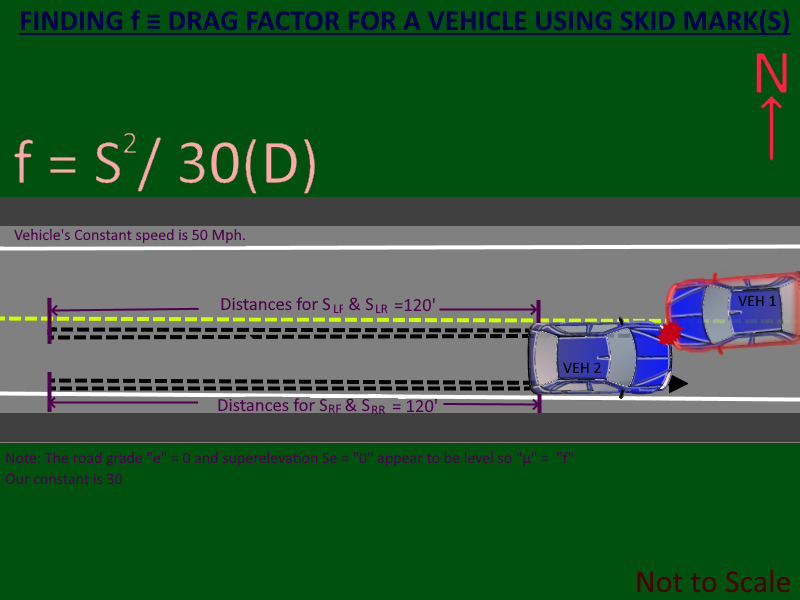So, We need to determine the Coefficient of Friction or the Drag Factor f.

We assume the speed of the vehicle is constant at 50 Mph. We've measured the measured the (Left Front Tire)SLF skid mark @ 120', the (Left Rear Tire)SLR skid mark @ 120', the (Right Front Tire)SRF skid mark @ 120', and the (Right Rear Tire)SRR skid mark @ 120'.

We get the average distance of the skid marks in feet DAvg' by getting the sum of all 4 skid mark distances SLF' + SLR' + SRF' + SRR' and then dividing the result by the number of skid marks (4) and we get an average measured Distance (D) of 120 feet'. Since all four tires were locked and skidding we have determined a 100% breaking efficiency and our road grade and super-elevation are "0".

DAvg.' = SLF + SLR + SRF + SRR / Number of Skid Marks measured.
DAvg'.DAvg.' = D = 120'

f = S2 / 30(D')
f = 502 Mph / 30(120')
f = 2500' / 3600'
f = 0.694
Note" we don't have a unit of measure for (f).

Special Note: Finding the f = Factor from skid marks like other formulas listed are derived from a more complex algebraic formulas. Being familiar with other formulas like the derivation of the minimum speed formula could help you visualize the mathematical progression.

Special Note: Finding D = Skid Distance (D) like other formulas listed are derived from a more complex algebraic formulas. Being familiar with these other formulas could help you visualize the mathematical progression.

. Last updated on (UTC-5/EST-Eastern Standard Time): May, 05-24-2022 10:28:36 AM.

##### Royalton Police Department
2460 VT RT 14
Royalton, VT 05068

Email: Help@RoyaltonPD.com
Phone: 802-763-7776
Dispatch: 802-234-9933
Fax: 802-763-7711